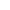Top | Introduction | Members | Activities | Call for Paper | Link |Japanese## "Design and Analysis of Practical Digital Signature Scheme"

 In this thesis, we focus on two kinds of digital signatures, that is, fast on-line signatures and proxy signatures. ``On-line'' means the phase of real-time requirement for signing message. The dominant factor of on-line phase usually consists of some modular arithmetics such as multiplication and modular reduction. A signature scheme without modular reduction is called ``on the fly'' signature. On the other hand, a proxy signature means the scheme, which allows a designated person to sign on behalf of an original signer. Our contributions are summarized as follows: - We research new mathematical problems, named the self powering RSA problem and the extended finding order problem. Those problems are used as the underlying problem in our schemes; - We propose a new on the fly signature by improving the Poupard-Stern scheme (PS-scheme). Our scheme has the efficiency in terms of both amount of work and transmitted data size; - We design and analysis an new fast on-line signature, which has new feature in that on-line multiplication is not required; - We construct new proxy signature schemes, which are based on the original signer's messa ge recovery. We also propose two practical schemes, whose security is based on Discrete logarithm and RSA problems, respectively. We investigate the above themes in terms of both security and efficiency. Our schemes satisfy the provable security using well-known or our proposed assumptions. All our schemes are enough practical from both computational and transmitted-data point of view. [ back ]